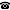# 2008 seminar talk: Computable uncountably categorical structures

Talk held by Ekaterina Fokina (KGRC) at the KGRC seminar on 2008-10-16.

### Abstract

Computable model theory hybridizes two branches of mathematical logic, namely, model theory and computability (recursion) theory. Given a suitable way to code mathematical objects into natural numbers, we can talk about effective properties of these objects. The main questions of computable model theory are the following. Let T be a first-order theory. Does T have a computable (recursive) model? If T has a computable model, what is the algorithmic complexity of T? Can we say something about the complexity of other models of T? Such questions are especially interesting for objects that are important from the model-theoretic point of view. In this talk we will discuss the known results and open questions for the class of uncountably categorical theories.

Kurt Gödel Research Center for Mathematical Logic. Währinger Straße 25, 1090 Wien, Austria.+43-1-4277-50501. Last updated: 2010-12-16, 04:37.